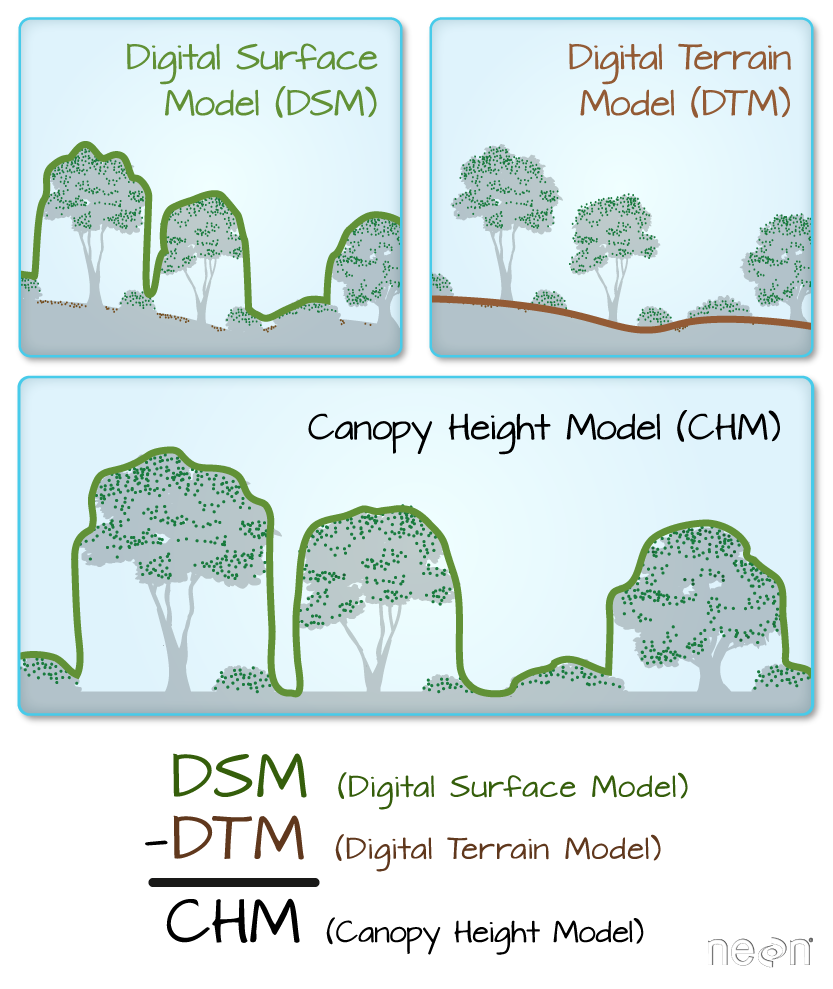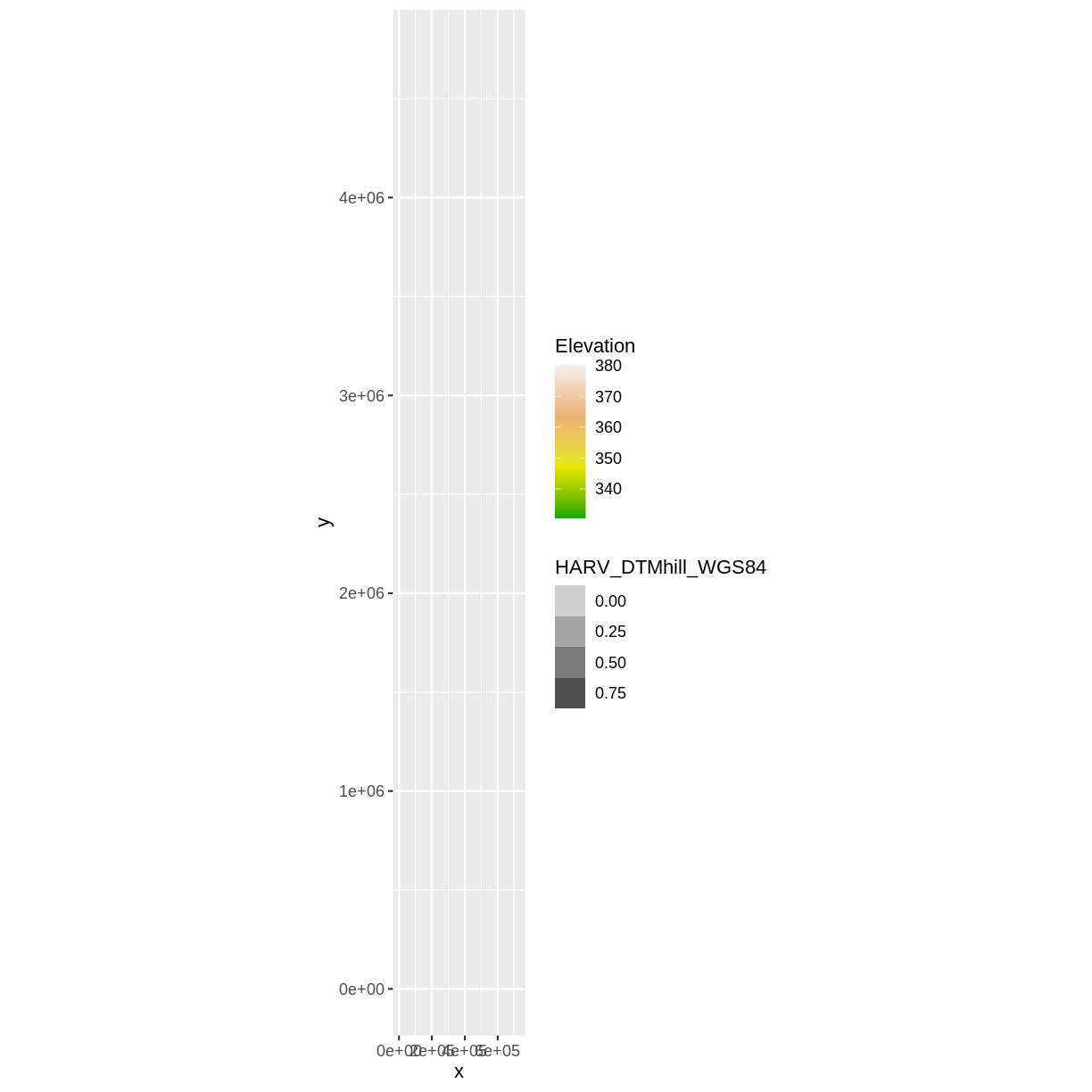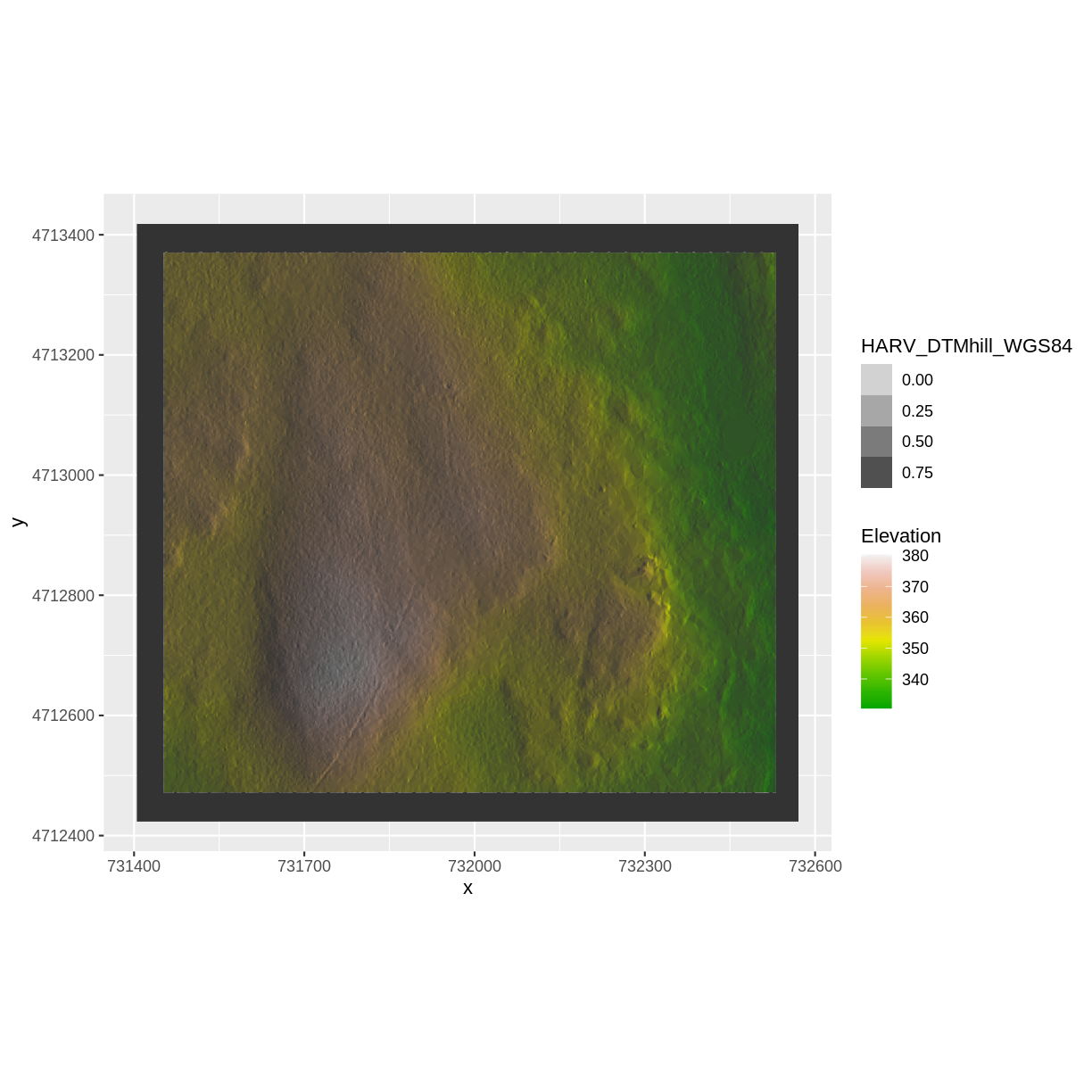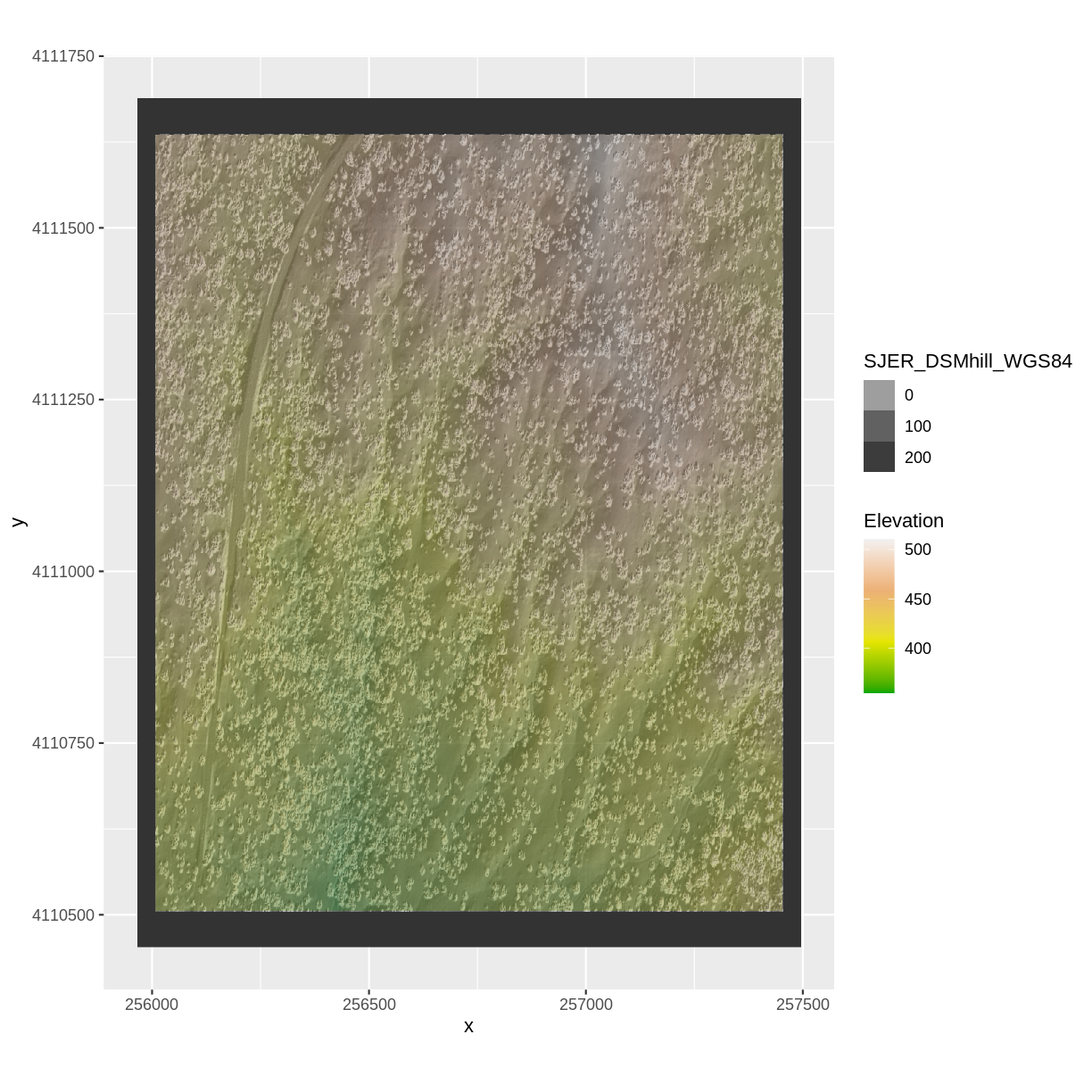This lesson is being piloted (Beta version)

# Reproject Raster Data in R

## Overview

Teaching: 40 min
Exercises: 20 min
Questions
• How do I work with raster data sets that are in different projections?

Objectives
• Reproject a raster in R.

## Things You’ll Need To Complete This Episode

See the lesson homepage for detailed information about the software, data, and other prerequisites you will need to work through the examples in this episode.

Sometimes we encounter raster datasets that do not “line up” when plotted or analyzed. Rasters that don’t line up are most often in different Coordinate Reference Systems (CRS). This episode explains how to deal with rasters in different, known CRSs. It will walk though reprojecting rasters in R using the `projectRaster()` function in the `raster` package.

## Raster Projection in R

In the Plot Raster Data in R episode, we learned how to layer a raster file on top of a hillshade for a nice looking basemap. In that episode, all of our data were in the same CRS. What happens when things don’t line up?

For this episode, we will be working with the Harvard Forest Digital Terrain Model data. This differs from the surface model data we’ve been working with so far in that the digital terrain model (DTM) includes the tops of trees, while the digital surface model (DSM) shows the ground level.

We’ll be looking at another model (the canopy height model) in a later episode and will see how to calculate the CHM from the DSM and DTM. Here, we will create a map of the Harvard Forest Digital Terrain Model (`DTM_HARV`) draped or layered on top of the hillshade (`DTM_hill_HARV`).##The Data

In this lesson, we will be working with two field sites: the Harvard Forest (`HARV`) and San Joaquin Experimental Range (`SJER`).

In this lesson, the rasters we will use are: `HARV_dtmCrop.tif` (DTM) and `HARV_DTMhill_WGS84.tif` (DTM - WGS84).

First, we need to import the DTM and DTM hillshade data.

``````DTM_HARV <- raster("data/raster/HARV_dtmCrop.tif")

DTM_hill_HARV <- raster("data/raster/HARV_DTMhill_WGS84.tif")
``````

Next, we will convert each of these datasets to a dataframe for plotting with `ggplot`.

``````DTM_HARV_df <- as.data.frame(DTM_HARV, xy = TRUE)

DTM_hill_HARV_df <- as.data.frame(DTM_hill_HARV, xy = TRUE)
``````

Now we can create a map of the DTM layered over the hillshade. For DTM, we specify that we want 10 terrain colors with the argument `terrain.colors(4)` inside the function `scale_fill_gradientn()`

``````ggplot() +
geom_raster(data = DTM_HARV_df ,
aes(x = x, y = y,
fill = HARV_dtmCrop)) +
geom_raster(data = DTM_hill_HARV_df,
aes(x = x, y = y,
alpha = HARV_DTMhill_WGS84)) +
scale_fill_gradientn(name = "Elevation", colors = terrain.colors(4)) +
coord_quickmap()
``````Our results are curious - neither the Digital Terrain Model (`DTM_HARV_df`) nor the DTM Hillshade (`DTM_hill_HARV_df`) are plotted.

If we look at the axes, we can see that the projections of the two rasters are different. When this is the case, `ggplot` won’t render the image. It won’t even throw an error message to tell you something has gone wrong. We can look at Coordinate Reference Systems (CRSs) of the DTM and the hillshade data to see how they differ.

``````crs(DTM_HARV)
``````
``````CRS arguments:
+proj=utm +zone=18 +datum=WGS84 +units=m +no_defs +ellps=WGS84
+towgs84=0,0,0
``````
``````crs(DTM_hill_HARV)
``````
``````CRS arguments:
+proj=longlat +datum=WGS84 +no_defs +ellps=WGS84 +towgs84=0,0,0
``````

`DTM_HARV` is in the UTM projection, with units of meters while `DTM_hill_HARV` is in `Geographic WGS84` - which is represented by latitude and longitude values.

Because the two rasters are in different CRSs, they don’t line up when plotted in R. We need to reproject (or change the projection of) `DTM_hill_HARV` into the UTM CRS. Alternatively, we could reproject `DTM_HARV` into WGS84.

## Reproject Rasters

We can use the `projectRaster()` function from the `raster` package to reproject a raster into a new CRS. Keep in mind that reprojection only works when you first have a defined CRS for the raster object that you want to reproject. It cannot be used if no CRS is defined. Lucky for us, the `DTM_hill_HARV` has a defined CRS.

## Data Tip

When we reproject a raster, we move it from one “grid” to another. Thus, we are modifying the data! Keep this in mind as we work with raster data.

To use the `projectRaster()` function, we need to define two things:

1. the object we want to reproject and
2. the CRS that we want to reproject it to.

The syntax is `projectRaster(RasterObject, crs = CRSToReprojectTo)`

We want the CRS of our hillshade to match the `DTM_HARV` raster. We can thus assign the CRS of our `DTM_HARV` to our hillshade within the `projectRaster()` function as follows: `crs = crs(DTM_HARV)`. Note that we are using the `projectRaster()` function on the raster object, not the `data.frame()` we use for plotting with `ggplot`.

First we will reproject our `DTM_hill_HARV` raster data to match the `DTM_HARV` raster CRS. We call this projected raster `DTM_hill_HARV_UTM` as it will now be projected in the UTM CRS from `DTM_HARV`:

``````DTM_hill_HARV_UTM <- projectRaster(DTM_hill_HARV,
crs = crs(DTM_HARV))
``````

Now we can compare the CRS of our new DTM hillshade and DTM HARV rasters to verify that they are now indeed in the same CRS.

``````crs(DTM_hill_HARV_UTM)
``````
``````CRS arguments:
+proj=utm +zone=18 +datum=WGS84 +units=m +no_defs +ellps=WGS84
+towgs84=0,0,0
``````
``````crs(DTM_HARV)
``````
``````CRS arguments:
+proj=utm +zone=18 +datum=WGS84 +units=m +no_defs +ellps=WGS84
+towgs84=0,0,0
``````

We can also compare the extent of the two objects.

``````extent(DTM_hill_HARV_UTM)
``````
``````class      : Extent
xmin       : 731404.9
xmax       : 732570.9
ymin       : 4712423
ymax       : 4713418
``````
``````extent(DTM_HARV)
``````
``````class      : Extent
xmin       : 731453
xmax       : 732531
ymin       : 4712472
ymax       : 4713370
``````

The extent values of `DTM_hill_HARV_UTM` and `DTM_hill_HARV` are very similar, but not quite identical. Why do you think this is?

## Challenge: Extent Change with CRS Change

Why do you think the two extents differ?

The extent for DTM_hill_HARV_UTM is in UTMs so the extent is in meters. The extent for DTM_hill_HARV was in lat/long so the extent was expressed in decimal degrees. When converting decimal degrees to meters, conversion is not directly one-to-one and therefore the extents are approximately equivalent.

## Deal with Raster Resolution

Let’s next have a look at the resolution of our reprojected hillshade versus our original data.

``````res(DTM_hill_HARV_UTM)
``````
`````` 1.000 0.998
``````
``````res(DTM_HARV)
``````
`````` 1 1
``````

These two resolutions are different, but they’re representing the same data. We can tell R to force our newly reprojected raster to be 1m x 1m resolution by adding a line of code (`res=`) within the `projectRaster()` function.

``````DTM_hill_HARV_UTM <- projectRaster(DTM_hill_HARV,
crs = crs(DTM_HARV),
res = 1)
``````

Let’s re-check our resolution:

``````res(DTM_hill_HARV_UTM)
``````
`````` 1 1
``````
``````res(DTM_HARV)
``````
`````` 1 1
``````

Now both our resolutions and our CRSs match, so we can plot these two data sets together. For plotting with `ggplot()`, we will need to create a dataframe from our newly reprojected raster.

``````DTM_hill_HARV_2_df <- as.data.frame(DTM_hill_HARV_UTM, xy = TRUE)
``````

We can now create a plot of this data.

``````ggplot() +
geom_raster(data = DTM_HARV_df ,
aes(x = x, y = y,
fill = HARV_dtmCrop)) +
geom_raster(data = DTM_hill_HARV_2_df,
aes(x = x, y = y,
alpha = HARV_DTMhill_WGS84)) +
scale_fill_gradientn(name = "Elevation", colors = terrain.colors(10)) +
coord_quickmap()
``````We have now successfully draped the Digital Terrain Model on top of our hillshade to produce a nice looking, textured map!

## Challenge: Reproject, then Plot a Digital Surface Model

Create a map of the San Joaquin Experimental Range field site using the `SJER_DSMhill_WGS84.tif` and `SJER_dsmCrop.tif` files.

Reproject the data as necessary to make things line up!

``````# import DSM
DSM_SJER <- raster("data/raster/SJER_dsmCrop.tif")
DSM_hill_SJER_WGS <-
raster("data/raster/SJER_DSMhill_WGS84.tif")

# reproject raster
DSM_hill_UTMZ18N_SJER <- projectRaster(DSM_hill_SJER_WGS,
crs = crs(DSM_SJER),
res = 1)

# convert to data.frames
DSM_SJER_df <- as.data.frame(DSM_SJER, xy = TRUE)

DSM_hill_SJER_df <- as.data.frame(DSM_hill_UTMZ18N_SJER, xy = TRUE)

ggplot() +
geom_raster(data = DSM_hill_SJER_df,
aes(x = x, y = y,
alpha = SJER_DSMhill_WGS84)
) +
geom_raster(data = DSM_SJER_df,
aes(x = x, y = y,
fill = SJER_dsmCrop,
alpha=0.8)
) +
scale_fill_gradientn(name = "Elevation", colors = terrain.colors(4)) +
coord_quickmap()
``````If you completed the San Joaquin plotting challenge in the Plot Raster Data in R episode, how does the map you just created compare to that map?

• Use the `projectRaster()` function to convert between CRSs.